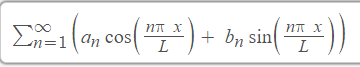# Math: $\displaystyle\sum_{n = 1}^\infty \left[a_n\cos\left(\dfrac{n\pi x}{L}\right)+b_n\sin\left(\dfrac{n\pi x}{L}\right)\right]$

2 posts / 0 new
Jeynard CaroMath: $\displaystyle\sum_{n = 1}^\infty \left[a_n\cos\left(\dfrac{n\pi x}{L}\right)+b_n\sin\left(\dfrac{n\pi x}{L}\right)\right]$

Evaluate:fitzmerl duronhmmm....Study Fourier Series first....hehehehehe

Fourier series equation yan. Yung mga complex (sawtooth waves, triangular waves at iba pa) at mga panget na mga wave shapes ay kayang ma-decompose into sine and cosine waves.

• Mathematics inside the configured delimiters is rendered by MathJax. The default math delimiters are $$...$$ and $...$ for displayed mathematics, and $...$ and $...$ for in-line mathematics.